﻿ Selection of a Significant Numerical Model of Plasticity for the Purpose of Numerical Analysis of Plastic ReinforcementPublications are Open
Access in this journal
Article Versions
Export Article
• Normal Style
• MLA Style
• APA Style
• Chicago Style
Research Article
Open Access Peer-reviewed

### Selection of a Significant Numerical Model of Plasticity for the Purpose of Numerical Analysis of Plastic Reinforcement

Jozef Bocko, Ingrid Delyová , Peter Sivák, Marián Tomko
American Journal of Mechanical Engineering. 2017, 5(6), 334-340. DOI: 10.12691/ajme-5-6-21
Published online: December 15, 2017

### Abstract

The paper is focused on the process of selecting a suitable material plasticity model. Such a model would be used in the future numerical analysis of the behaviour of selected structural elements in the elastic-plastic deformation of the steel in the SolidWorks software environment. These analyzes should include monitoring the behaviour of the material under the conditions of its plastic reinforcement (strain - hardening). Numerical modelling used two models of plasticity, namely the von Mises and Tresca's models. Subsequently, a comparison of the match between the real load-deformation diagram, possibly stress-strain diagram, obtained by the experimental static tensile test and the diagram obtained from the numerical simulation of two different steel-based structural materials was performed. The results of the dual tensile test analysis showed that the von Mises model achieves greater consistency with the tensile curve based the actual tensile test. Therefore, this model will be later used in subsequent numerical simulations of the material under the conditions of its plastic reinforcement.

### 1. Introduction

As part of the numerical analysis of the behaviour of selected structural elements in the elastic-plastic area of deformation in the SolidWorks software environment, a material analysis is planned under the conditions of its plastic reinforcement. In the SolidWorks program environment, a particular chosen model of plasticity must then be applied. However, this program, like similar FEM program products, offers several models of plastic behaviour of material 1, 2. The decision on which of the models is most relevant to reality and which will be applied to a later numerical analysis can be made by comparing of the results of independent analyzes. The choice of a significant plasticity model for the numerical analysis will be performed on the basis of actual and virtual tensile tests, followed by a comparison of the experimental and numerical stress-strain diagrams.

### 2. Plasticity Criteria

2.1. The Transition from Elastic to Plastic Deformation

The deformations of the body in the elastic state are clearly described by the functions of the stress vector components. In case of uniaxial stress, the elastic state criterion can be written by this scalar inequality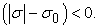(1)

The quantity σ0 here corresponds to the critical stress value, e.g. yield point.

In the case of the transition from elastic to plastic, the relevant plasticity criterion can be written in a scalar shape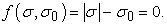(2)

In general triaxial stress, where the stress vector is determined by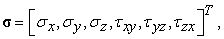(3)

the relevant criterion of plasticity is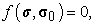(4)

respectively for material constants ki the condition is in shape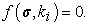(5)

There are currently a number of hypotheses and plasticity criteria based on different criteria. Accepted and often used include, for example, the von Mises, Tresca, Mohr-Coulomb, Drucker-Prager and others.

2.2. The Von Mises Criterion of Plasticity

The von Mises criterion (Huber-Mises-Hencky) is based on the potential energy of stress for shape change as well as the equivalent yield criterion of strength. The condition of plasticity itself is based on the hypothesis that in the plastic state, the shear stress intensity τi is constant and equal to the critical shear stress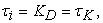(6)

where shear stress intensity is equal to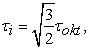(7)

or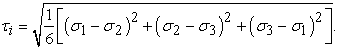(8)

For uniaxial stress and for yield stress Re there is a relationship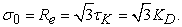(9)

The resulting condition of the von Mises plasticity criterion can be expressed by means of principal stresses in the shape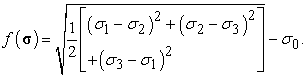(10)
2.3. Tresca's Criterion of Plasticity

Under this criterion, it is assumed that the plastic transformation begins at the moment when the maximum shear stress τmax (σ) reaches a critical and constant value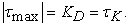(11)

The relevant hypothesis was, for the first time, pronounced by Saint-Venant. This theory was completely proven by Tresca. Explaining this condition is more complex if it is not clear which of the three extreme shear stresses is maximum.

The maximum shear stresses in the individual principal planes can be gradually expressed in shape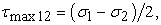(12)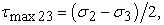(13)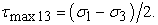(14)

At the same time, for uniaxial stress there is a relationship:(15)

The resulting condition of Tresca's plasticity criterion then can be expressed by means of the largest difference of the principal stresses in the shape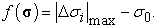(16)

The criterion of the constant value of the maximum shear stress is less accurate than the criterion of the constant value of the shear stress intensity. However, because of its simplicity it is used more often.

2.4. Graphical Expression of Plasticity Criteria

The general criterion of plasticity can be expressed by the surface of plasticity in the area determined by the Cartesian coordinates of the principal stresses σ1, σ2 and σ3. This area divides the stress area into two parts. For the first of these, f < 0, i.e. the criterion of plasticity is not fulfilled, and for the other of the fields f > 0, i.e. the plasticity criterion is satisfied. On their interface there is a boundary area - the surface of plasticity, for which f = 0. If this area is unchanged and there is no change in tension in the process of loading, relieving and reloading, then it is the initial surface in the area. Such surface corresponds to an ideal plastic material.

If material is considered, whose plasticity area is not affected by medium stress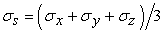(17)

and while being isotropic, the area of plasticity in the three-dimensional space of the main stresses σ1, σ2 and σ3. is geometrically represented by a cylindrical surface whose axis is identical to the so-called hydrostatic axis. The hydrostatic axis is a straight line passing through the origin of the coordinate system. This axis is also diverted from the directions for σ1, σ2 and σ3. The points for this axis can be expressed by relationship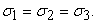(18)

The plane perpendicular to the hydrostatic axis, which at the same time passes the origin of the coordinates, is so-called deviatoric plane. This one is then determined by the relationship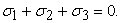(19)

The surface of the plasticity and the deviator plane then defines the plasticity curve. This can then have a various numbers of symmetry axes, depending on the behaviour of the material or the applied plasticity criterion.

• Figure 1. Surfaces of the plasticity for the von Mises and Tresca's criteria of plasticity
• Figure 2. Deviatoric sections for the von Mises and Tresca's criteria of plasticity
• Figure 3. Biaxial stress for the von Mises and Tresca's criteria of plasticity

The von Mises criterion of plasticity can be expressed in the form of an infinitely long cylinder and the Tresca's criterion of plasticity in the form of an infinitely long regular six-angular prism according to Figure 1. The axes of both surfaces are identical to the hydrostatic axis. A common graphical representation of both conditions by the deviatoric sections is in Figure 2 and a common graphical representation for biaxial stress is shown in Figure 3. 3, 4, 5, 6

### 3. Experimental Static Tensile Test

Two specimens, were made from two different steels and were subjected to complete tensile test, that is until the material was broken down. The material of the first test specimen (Figure 4), HS 001/17, was stainless steel S355J2C+N suitable for cold forming. The material of the second specimen (Figure 5), bearing the HS 002/17 designation, was the noble 42CrMo4 structural steel. This steel is used for more stressed machine parts where high strength and high toughness are required.

The static tensile test of materials was performed according to the STN EN 10002-1 standard on a universal testing machine Herkules with a maximum force of 40 kN.

• Figure 4. Model and the real specimen HS 001/17
• Figure 5. Model and the real specimen HS 002/17
• Figure 6. Load-deformation (tensile) diagram of the specimen HS 001/17
• Figure 7. Load-deformation (tensile) diagram of the specimen HS 002/17

The resulting load-deformation diagrams are in Figure 6 a Figure 7. In Figure 6 is the load-deformation diagram of HS 001/17 and in Figure 7 is the load-deformation diagrams of sample 002/17. It is evident from tensile diagrams that the S355J2C+N steel has a significant yield point, but the 42CrMo4 steel has no that value. In this case, so-called conventional value of yield point is used. This value represents a stress at which the permanent deformation reaches 0.2% (ε = 2·10-3) of the initial value. The yield strength of the first specimen is 405 MPa, and the second specimen has a value of 803 MPa. In the case of S355J2C+N steel, the ultimate strength is 558 MPa, and in the case of 42CrMo4 steel, the ultimate strength is 997 MPa.

The curves of the tensile diagram have linear character in the area of elastic deformation, i.e. below the yield point. However, for specimen HS 001/17, the acquired dependence is initially curled. The reason for this phenomenon was the sliding of the specimen in the jaws of the testing machine at the beginning of the test.

### 4. Simulated Static Tensile Test

A simulated static tensile test was performed in the SolidWorks program environment. The simulation of the load was realized only until the maximum stress equal to the ultimate strength. The virtual specimens were modelled according to the actual shapes and sizes of specimens used in the experimental tensile test, which are also subject to STN EN 10002-1. However, the FEM numerical system did not offer specimen materials. Therefore, the properties of individual materials were deduced from technical cards 7, 8. Due to the testing of both materials in the plastic area, it was also necessary to obtain stress-strain curves of the dependence between stress and the normal strain of individual materials. The material curve of S355J2C+N defined in the program is in Figure 8 and the material curve of 42CrMo4 is in Figure 9. The individual points of these curves were determined from the tensile diagrams of the test specimens.

The values in the stress-strain curves were transformed only until the ultimate strength of both materials for the von Mises plasticity model. Subsequently, according to Figure 10 for the samples, a finite element network was created. Also, boundary conditions and load have been defined.

• Figure 8. Stress-strain curve of the S355J2C+N
• Figure 9. Stress-strain curve of the 42CrMo4
• Figure 10. Finite element network, boundary conditions and load of virtual models of the specimens HS 001/17 and HS 002/17

### 5. The Results of Numerical Modelling

5.1. For the Von Mises Model

In Figure 11 and Figure 12, the results of simulations of virtual specimens HS 001/17 and HS 002/17 are shown. The distribution of the reduced stresses according to the von Mises yield criterion is shown. The von Mises material model of plasticity was used.

• Figure 11. Result of simulation for HS 001/17 and the von Mises model
• Figure 12. Result of simulation for HS 002/17 and the von Mises model

The results of these simulations have been further utilized for forming the stress-strain diagrams shown in Figure 13 and Figure 14. The corresponding values of the reduced stresses and normal strains were read at the point halfway along the length of the virtual model.

• Figure 13. Virtual stress-strain diagram for HS 001/17 and the von Mises model
• Figure 14. Virtual Stress-strain diagram for HS 002/17 and the von Mises model
5.2. For Tresca Model

In Figure 15 and Figure 16, the results of further simulations of virtual specimens HS 001/17 and HS 002/17 are shown. The distribution of the reduced stresses again according to the von Mises yield criterion is shown. However Tresca material model of plasticity was used.

• Figure 15. Result of simulation for HS 001/17 and Tresca model
• Figure 16. Result of simulation for HS 002/17 and Tresca model

The results of these simulations have been further utilized for forming the stress-strain diagrams shown in Figure 17 and Figure 18. The corresponding values of the reduced stresses and normal strains were read at the point halfway along the length of the virtual model.

• Figure 17. Virtual stress-strain diagram for HS 001/17 and Tresca model
• Figure 18. Virtual stress-strain diagram for HS 002/17 and Tresca model

### 6. Comparison of the Experimental and Numerical Analysis Results

The results of the experimental tensile tests according to Figure 6 and Figure 7 and the results of the simulated tensile tests according to Figure 13, Figure 14, Figure 17 and Figure 18, were then compared. It was mainly about the level of conformity, respectively about the differences in the results obtained.

6.1. For the Von Mises Model

By comparing of the respective segments of according to Figure 6 and Figure 13 for samples HS 001/17 in Figure 19 it has been found, that the curves are practically identical in the elastic area. There was a certain minimum deviation in the plastic area. Main tracking values, i.e. the yield strength and the ultimate strength are the same.

• Figure 19. Comparison of the experimental and numerical analysis results - von Mises (HS 001/17)

By comparing of the respective segments of according to Figure 7 and Figure 14 for samples HS 002/17 in Figure 20 it has been found, that the curves are not exactly identical in the elastic area. Observed slight variations can be explained by the fact that the elastic part of the experimental tensile diagram is not entirely linear. There were much greater variations in the plastic area. Despite these findings, the yield strength and the ultimate strength are practically comparable.

• Figure 20. Comparison of the experimental and numerical analysis results - von Mises (HS 002/17)
6.2. For Tresca Model

By comparing of the respective segments of according to Figure 6 and Figure 17 for samples HS 001/17 in Figure 21 it has been found, that the curves are not exactly identical in the elastic area, but the differences are not large. But there was a significant deviation in the plastic area. The value of the yield strength is the same, but the ultimate strength varies. The difference is a few MPa. Deviations are larger than for the von Mises model.

• Figure 21. Comparison of the experimental and numerical analysis results - Tresca (HS 001/17)

By comparing of the respective segments of according to Figure 7 and Figure 18 for samples HS 002/17 in Figure 22 it has been found, that the curves are not exactly identical in the elastic area. Slight variations in the range up to 110 kN can be explained by the fact that the elastic part of the experimental tensile diagram is not entirely linear. There are much bigger deviations in the area above 110 kN and also in the plastic area. The values of the yield strength and the ultimate strength are roughly the same.

• Figure 22. Comparison of the experimental and numerical analysis results - Tresca (HS 002/17)

### 7. Conclusion

For dual tensile analysis - experimental and numerical, two materials were used. They were chosen so that one material had a significant yield point and the other material did not. The values of the yield strength and the ultimate strength were also different for the individual materials. Numerical modelling used two models of plasticity, namely von Mises and Tresca. A comparison of the match between the real tensile diagram obtained by the experimental static tensile test and the diagram obtained by the numerical simulation was then made. The results obtained show that the von Mises model of the plasticity compared to the Tresca model achieves greater consistency with the tensile curve from the experimental tensile test. Therefore, this model of plasticity will be later used in subsequent numerical simulations in the elastic-plastic area for material analyzes under conditions of its plastic reinforcement.

### Acknowledgements

This work was supported by the Ministry of Education of Slovakia Foundation under Grant project VEGA No. 1/0393/14, project VEGA No.1/0731/16.

### References

  J. Bocko and Š. Segľa, Numerické metódy mechaniky tuhých a poddajných telies, TU Košice, 2016, 248 p. ISBN 978-80-553-3065-5. In article PubMed  V. Ivančo and R. Vodička, Numerické metódy mechaniky telies a vybrané aplikácie, TU Košice, TypoPress Košice, 2012, 354 p. ISBN 978-80-553-1257-6. In article  R. Servít, E. Doležalová and M. Crha, Teorie pružnosti a plasticity I, SNTL Praha, Alfa Bratislava, 1981, 456 p. In article  S. Kaliszky, Plasticity, Akadémiai Kiadó Budapest, 1989. In article  F. Trebuňa and F. Šimčák, Odolnosť prvkov mechanických sústav, TU Košice, Emilena Košice, 2004, 980 p. ISBN 80-8073-148-9. In article  J. Lubliner, Plasticity Theory, Macmillan Publishing Company, New York, 1990. In article PubMed  http://www.lucefin.com/wp-content/files_mf/42crmo4astmen.pdf. In article View Article  http://www.lucefin.com/wp-content/files_mf/06s355j2ing45.pdf. In article View Article

Published with license by Science and Education Publishing, Copyright © 2017 Jozef Bocko, Ingrid Delyová, Peter Sivák and Marián TomkoThis work is licensed under a Creative Commons Attribution 4.0 International License. To view a copy of this license, visit http://creativecommons.org/licenses/by/4.0/